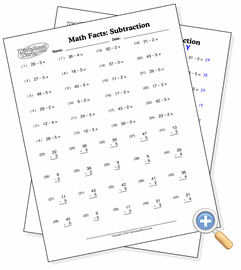# Subtraction from FiftyDevelop subtraction skills starting from numbers up to fifty

This worksheet presents subtraction problems that start with values as high as fifty. The answer to each problem will be determined by which numbers you want to subtract with so that the result is never below zero.